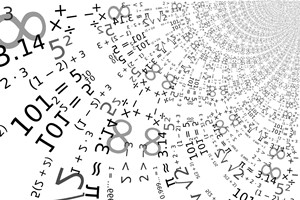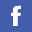## MATH

Home > Undergraduate > Courses Information and Outline > MATH Courses | GE Courses | University Core | Cross Institutional Course
MATH Level 1
• MATH 1005 Calculus I
• MATH 1017 Mathematics for Personal Financial Management
• MATH 1025 Understanding Mathematics and Statistics
• MATH 1026 Probability and Statistics with Software
• MATH 1205 Discrete Mathematics
MATH Level 2
• MATH 2005 Calculus, Probability, and Statistics for Computer Science
• MATH 2006 Calculus, Probability, and Statistics for Science
• MATH 2007 Programming with Mathematical Software
• MATH 2205 Multivariate Calculus
• MATH 2206 Probability and Statistics
• MATH 2207 Linear Algebra I
• MATH 2215 Mathematical Analysis
• MATH 2216 Statistical Methods and Theory
• MATH 2225 Calculus II
MATH Level 3
• MATH 3095 Summer Research I
• MATH 3096 Summer Research II
• MATH 3205 Operations Research I
• MATH 3206 Scientific Computing I
• MATH 3405 Differential Equations I
• MATH 3406 Abstract Algebra
• MATH 3407 Linear Algebra II
• MATH 3415 Vector Calculus
• MATH 3416 Complex Analysis
• MATH 3426 Number Theory
• MATH 3427 Real Analysis
• MATH 3495 Job Practicum I
• MATH 3496 Job Practicum II
• MATH 3497 Job Practicum III
• MATH 3605 Numerical Methods II
• MATH 3606 Differential Equations II
• MATH 3615 Introduction to Imaging Science
• MATH 3616 Scientific Computing II
• MATH 3626 Computational Statistics for Data Science
• MATH 3805 Regression Analysis
• MATH 3806 Multivariate Statistical Methods
• MATH 3807 Simulation
• MATH 3815 Statistical Design and Analysis of Experiments
• MATH 3816 Statistical Analysis of Sample Surveys
• MATH 3825 Life Insurance and Life Contingencies
• MATH 3826 Operations Research II
• MATH 3836 Data Mining
• MATH 3845 Interest Theory and Applications
MATH Level 4
• MATH 4205 Topics in Probability Theory and Stochastic Processes
• MATH 4207 Computational Finance
• MATH 4216 Mathematical Finance
• MATH 4225 Foundation of Big Data and Learning
• MATH 4226 Introduction to Deep Learning
• MATH 4227 Programming for Data Science
• MATH 4465 Special Topics in Mathematics I
• MATH 4606 Functional Analysis
• MATH 4615 Numerical Linear Algebra
• MATH 4805 Applied Nonparametric Statistics
• MATH 4807 Categorical Data Analysis
• MATH 4815 Interior Point Methods for Convex Optimization
• MATH 4816 Optimization Theory and Techniques
• MATH 4817 Stochastic Models
• MATH 4825 Survival Analysis
• MATH 4826 Time Series and Forecasting
• MATH 4837 Risk and Portfolio Management
• MATH 4998 Mathematical Science Project I
• MATH 4999 Mathematical Science Project II### BSc (Hons) in Mathematics and Statistics

The programme is designed to provide students with a solid foundation in mathematical and statistical concepts with an aim towards developing logical thinking and analytical skills.Special emphasis on applications in industrial and commercial areas is stressed, and students are well trained to solve real-life problems in different areas.### Café Mathématique

Current students can join orientation and meet our alumni.

Details### Students Activities

Every year we have organized different activities for students.